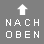## Example of an oracle laying with coins

Take three coins and toss them simultaneously six times. Note down the result.

The first throw determines the bottom line, the second throw determines the second last line, and so forth. For example, your result could look like this:
 Throw 6 5 4 3 2 1 Value H H T H T H Value T H T T T T Value H H H T T H If you throw heads, it counts three points and tails counts two points. Add the points. For each threefold throw the values could be 6,7,8, or 9 Points 8 9 7 7 6 8 Graded numbers are symbolised by a broken line and ungraded numbers by a solid line. First hexagram _ _ ___ ___ ___ _ _ _ _ The lines with a score of 6 or 9 are converted, i.e. 2 conversions were made here. In the example, these are lines two and five. If no 6 or 9 was thrown, no conversion takes place. Second hexagram _ _ _ _ ___ ___ ___ _ _

The first hexagram at the top has the meaning of 31 – Influence (Wooing)
The second hexagram has the meaning of 32 – DurationYou find the meaning by clicking on the calculated hexagram. There are the following 64 hexagrams:

Total 0 posts